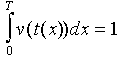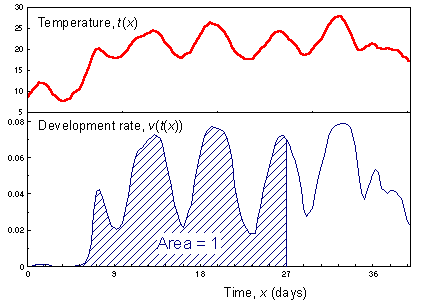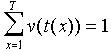8.6. Physiological time

What is time? This is the most fundamental question in science. It attracted the best brains in human history from St. Augustin to Einstein. What is the relationship between time and dynamics? Do we measure dynamics relative to time, or may be time should be measured with dynamics? Newtonian physics considered absolute and universal time which was above nature. Alternative point of view is that each system has its own individual time measured by the number of events. For example, time in human life can be measured by the number of decisions made. A person who lives passively, and avoids making decisions, remains a child psychologically even if he is biologically an adult. Further discussion on the problem of biological time can be found in the paper of Sharov (1995).

We can apply the concept of biological time to the development of poikilothermous organisms. The progress in organism's development can be viewed as a biological clock that measures physiological time. Physiological time goes fast when it is warm and slow when it is cold. Then, development rate can be defined as advance of physiological time per unit of calendar time. For example, if development rate of insect larvae is 0.07 per day then, taking the entire physiological time at larval stage as 100%, in one day the insect will increment its physiological time by 7%.

To determine the duration of development we will accumulate development rates rather than effective temperatures. Development completes on the day when accumulated development rates reach 1 (=100%), i.e., the following equation is true:where v(t) is the rate of development as a function of temperature, t; t(x) is temperature as a function of time, x; and T is the development time. If temperature is defined as a function of time (see the graph below), then at each time the rate of development is estimated as a non-linear function of time. Finally the integral of function v(t(x)) (the area under the curve) from 0 to T should be equal to 1.The discrete-time version of the previous equation is:How to measure physiological time?

There is no mechanical watch that measures physiological time. Also there are no hour and minute hands inside an organism. However, in some cases it is possible to find indicators of physiological time, e.g., concentration of hormones and metabolites, CO2 emission rate, etc. However, these indicators are usually not very accurate. For example, the respiration rate is high in gypsy moth eggs for ca. 2 weeks after oviposition; then it decreases and stay at low level. Thus, it is possible to distinguish between these two periods of egg development.

The problem of measurement can be evaded by preparing organisms of specific physiological age for the experiment. This is analogues to quantum mechanics where it is impossible to measure exact location of an electron without changing its momentum. But it is possible to prepare electrons with specific characteristics. To prepare organisms in a specific physiological age, we need to keep them in standard conditions for a specific time. For example, if the duration of insect pupal stage is 10 days in 25°, then after 5 days in this temperature, pupae will be in the middle of their pupal stage.

What are the advantages of physiological time concept?

As compared to the degree-day model, the concept of physiological time has the following advantages:
• It makes no arbitrarily assumptions on the shape of development rate function. This function can be linear, logistic, normal distribution, etc.
• It admits the effect of other factors besides temperature on the rate of development. The rate of development may depend on food quality and quantity, photoperiod, and other factors.
Before the concept of physiological time was developed there were attempts to incorporate the effect of factors other than temperature into the degree-day model. It was assumed that additional factors change the amount of degree-days required to complete development. For example, gypsy moth larvae develop faster on preferred tree species (oaks) as compared to non-preferred species (e.g., maples). It was assumed that more degree days should be accumulated by gypsy moth larvae on maple than on oak trees to pupate. This model does not work if larval migration is considered among trees because degree-days accumulated on different tree species are not comparable. For example, if larvae move from an oak tree to a maple tree, they become "younger". If they were ready to pupate on oak before migration, then after migration to maples they still have to accumulate additional degree-days to pupate.

Alexei Sharov 12/5/98